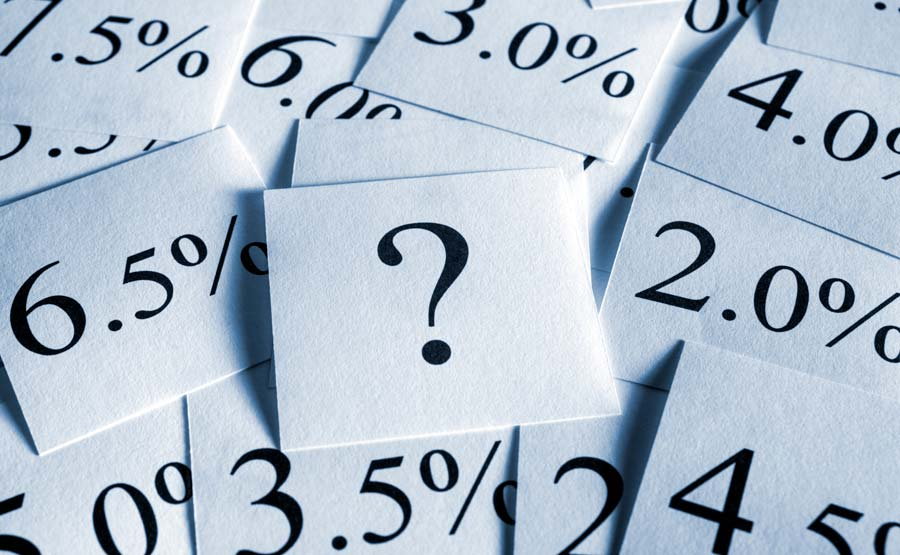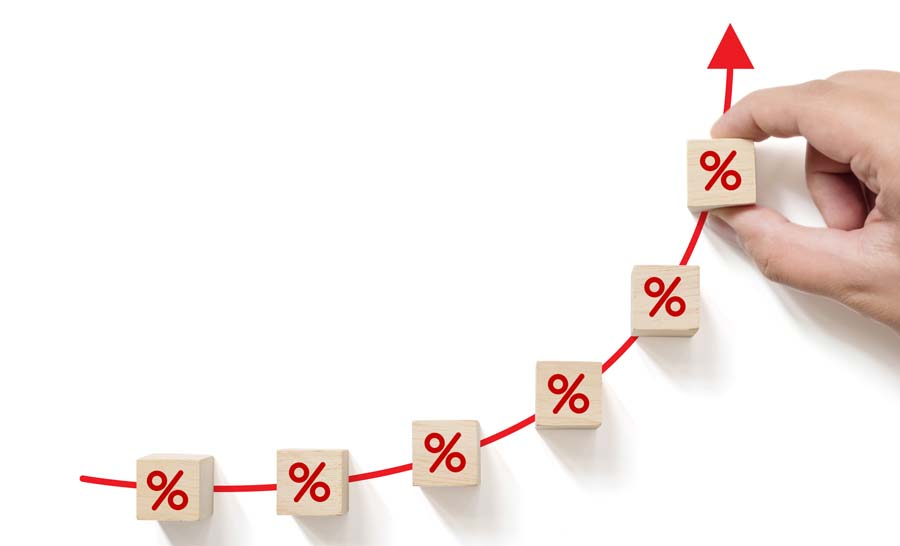# What is the Difference Between Nominal, Effective and APR Interest Rates?

| Last update: 13 November 2022

Whether you're paying interest on a debt or earning interest on savings and investments, the nominal interest rate is the figure used before considering inflation. Nominal interest rates are the ones advertised on financial products, but once they are adjusted for inflation, these can go up or down in real terms.

Compounding is the snowballing of interest on an investment or debt. When the accrued interest is added to the original sum, the combined total can then earn more interest, making compound interest essentially 'interest on interest'. The effective rate is how much interest you will really owe or receive once compounding is considered. APR is the annual percentage rate: the total amount of interest you pay on a borrowed sum per year.## What is nominal interest rate?

You'll see nominal interests rates advertised everywhere, from the posters for attractive savings accounts to the flyers advertising the rates of credit cards. Nominal interest rates are also sometimes called the 'base rate'.

Nominal interest does not include changes for inflation, however, and once inflation is adjusted for, the actual interest rate will be different.

Inflation is the process by which the same goods and services become more expensive over time (think: your grandparents complaining that a can of Coke once cost 20 cents and is now \$2).

If you think of the savings account or the credit card as a product, then just like a can of Coke, it makes sense that it would also become more expensive over time. The nominal interest rate is directly influenced by inflation, and it grows accordingly.

This can be a good thing for the consumer when it's an investment (more interest being paid to you than you first thought) or a nuisance when you've borrowed (as you'll end up owing more than you were expecting). Don't accept this rate at face value!

## What is compounding?

Compounding is the snowball effect of interest. A small snowball can't gather much new snow when you roll it; there's not enough of a base for it to stick to. A larger snowball, however, gathers lots of snow when you roll it, as there's so much more surface to grab.

The same principle applies to interest rates. If you put \$100 in a savings account with a 2% interest rate, then it will become \$102. But the following year, your 2% interest will be paid on the original sum (the principal \$100) PLUS accrued interest. 2% of \$102 is \$2.04, so the compound interest accrued is 4 cents more than the interest paid on the same principal deposit the previous year.

The year after that, compound interest would be paid on the new total, which stands at \$104.04, putting the third year's interest payment up to \$2.08, bringing the bank balance to \$106.12. It will continue to increase by a greater amount in every compound period, even when no further deposits are made by the investor.

• Year 1: \$100 at 2% = \$102 total.
• Year 2: \$102 at 2% = \$104.04 total.
• Year 3: \$104.04 at 2% = \$106.12 total.

Again, compound interest is a positive thing when you're saving and investing, because you earn more. If you've borrowed money with a credit card or loan, though, compound interest can be a cause for concern. The faster you repay a debt, the less compound interest you have to pay.## What is the effective rate?

The effective rate of interest is the actual amount of interest paid or earned over time, after compounding is considered. It's sometimes called the market rate of interest, annual percentage yield (APY), or even the yield to maturity (which, yes, sounds quite like a euphemism for giving up the anti-ageing moisturiser).

### Here's an example:

Starfish Bank might advertise a loan with a nominal interest rate of 10%, where the compound interest is calculated monthly. Dolphin Bank could also have a 10% rate loan, but their compound interest is charged annually. A 3-year loan with Dolphin Bank would be cheaper to repay in total, because the compound interest has been calculated less often: their snowball hasn't been 'rolled' as many times as the monthly calculation with Starfish Bank. Although they both have a nominal rate of 10%, the effective rate of Dolphin Bank is better than Starfish Bank, because compounding has a lesser impact.

Considering the effective interest rate is, therefore, more useful than the nominal rate when comparing financial products.

## What is APR?

APR, or Annual Percentage Rate, is the most straightforward way to compare different loans, credit cards and mortgages.

APR is the amount of interest repaid in a year and can be expressed, like other interest rates, as either a nominal or effective rate. APR also takes into account for any fees or additional costs associated with the loan.

The nominal APR is the 'base rate' you would repay over a year (not factoring in inflation or compounding). For example, a car loan which charges 1% interest each month has a nominal APR of 12%. The effective APR adjusts for compounding, so that the same car loan might actually have an effective APR of 17.9% once the snowball effect is considered.

The Truth in Lending Act oversees how US lenders calculate their APR, recording fees and charges to ensure a transparent and fair system.

## Example of nominal, effective and APR rates

Let's give a simple example. Let's say that you've taken out a loan of \$10,000 over 5 years at a 5% interest rate, compounded monthly, with a \$200 setup fee. Your figures might look like this:

Nominal interest rate: 5%
Effective interest rate: 5.12%
APR (nominal): 5.82%

It's good to know your onions when it comes to borrowing and investing, so be sure to use our interest rate calculator to get your numbers right!